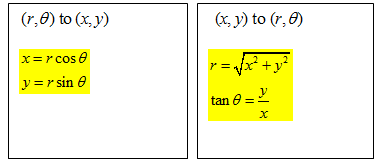# A New Way to Locate Points

## Objective

SWBAT convert from polar coordinates to rectangular or vice versa.

#### Big Idea

Instead of left and right directions, angle measures and radii can also specify a location.

## Launch

5 minutes

When we looked at rotated conic sections in an earlier lesson, my students realized that the point (1,1) could also be thought of as a rotation of 45 degrees and a distance of sqrt(2) from the origin. I remind students of this on this worksheet and use this as an introduction to polar coordinates. I outline my teaching strategies in the video below.

Unable to display content. Adobe Flash is required.

## Explore

20 minutes

Once students understand the premise behind polar coordinates, I will have them complete the table on the worksheet and brainstorm generalizations about the conversions from polar coordinates to rectangular and vice versa.

I encourage students to sketch out graphs for each one and to make right triangles. That will establish the strong connection to trigonometry. As students are working I will circulate and see how they are doing. Here is a list of things I will look for:

1. Are students using Pythagorean Theorem to find the r value?
2. If students are using inverse tangent to find the angle measure, is it in the right quadrant?
3. Are students making an overgeneralization that inverse tangent will always give the correct angle when it really only works in quadrants I and IV?
4. Are students sketching the locations of the points correctly?

## Share and Summarize

20 minutes

Once students have had time to brainstorm, I will have students share their thoughts about these conversions with the class. I find that they usually have an easier time converting from polar to rectangular, than from rectangular to polar. Here are the conversions we are looking for:It is important that students realize that the tan(θ) = y/x conversion is not perfect; they will have to think about the quadrant that the point is in and adjust the point accordingly.

The remainder of the worksheet provides an opportunity for students to graph a few points on the polar axis and to predict what some simple polar equations will look like. I encourage students to list points that satisfy each equation if they do not know what it will look like. That is usually a helpful strategy for them to envision the graph. We will share out our thoughts after they have a chance to think about it.

Finally, I will assign some problems from our textbook with polar coordinates. I will stick to problems that focus on single points or very simple graphs. We will work on more complicated equations as we progress in this unit.